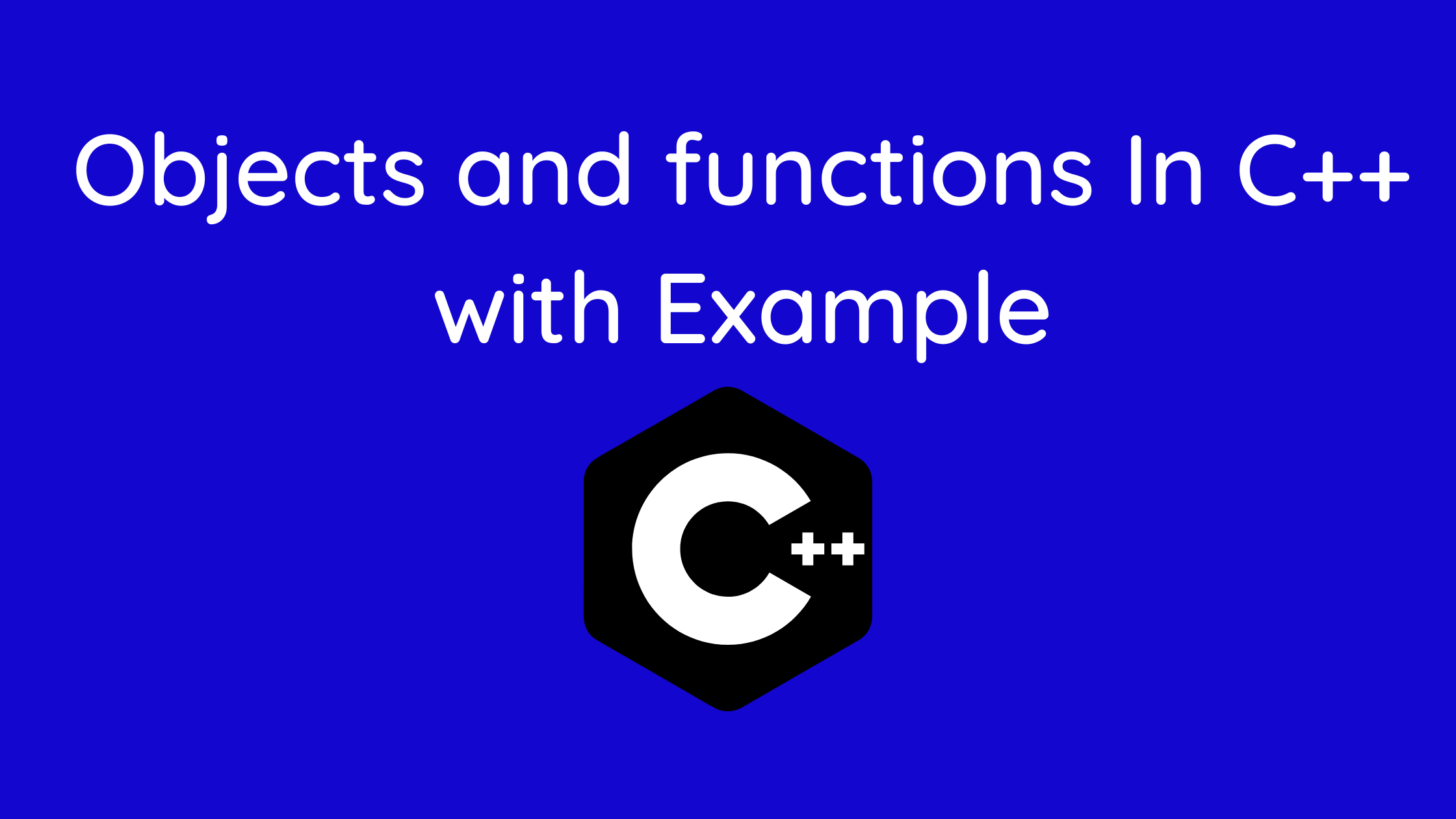## Objects and functions In C++

In C++, class objects can be passed as arguments and returned from functions in the same manner as other variables. No unique keyword or header file is necessary for this.

This tutorial will teach you how to provide objects to functions and get objects back from functions in C++.

Contents

## Passing an Object as an argument

In the same way, we call a function for other variables, we write the object name as the argument when passing an object as a parameter.

Syntax:

`function_name(object_name);`

## Example 1 Of C++ Pass Objects to Function

Similar to passing a structure to a function, we can also pass an object. In this case, the class A object is passed to the function disp() in class A. In the same manner, we can pass an object from one class to a function from another class.

```#include <iostream>
using namespace std;
class A {
public:
int age = 20;
char ch = 'A';

// function that has objects as parameters
void display(A &a) {

cout << "Age = " << a.age << endl;
cout << "Character = " << a.ch << endl;

}
};
int main() {
A obj;
obj.display(obj);
return 0;
}```

## Output

Age = 20
Character = A

We can give objects to a function in C++ in a similar way to how we pass ordinary parameters.

## Example 2 Of C++ Pass Objects to Function

```// C++ program to calculate the average marks of two students

#include <iostream>
using namespace std;

class Student {

public:
double marks;

// constructor to initialize marks
Student(double m) {
marks = m;
}
};

// function that has objects as parameters
void calculateAverage(Student s1, Student s2) {

// calculate the average of marks of s1 and s2
double average = (s1.marks + s2.marks) / 2;

cout << "Average Marks = " << average << endl;

}

int main() {
Student student1(88.0), student2(56.0);

// pass the objects as arguments
calculateAverage(student1, student2);

return 0;
}```

## Output

Average Marks = 72

## Returning an Object from a Function

In this example, there are two functions: display() accepts the Student object as an argument, and input() returns the Student object.

## Example 1 Of C++ Return Object from a Function

We employ the static cast operator to output the contents of a void pointer. The pointer is changed from being of type void* to the appropriate data type for the address it is storing:

```#include <iostream>
using namespace std;
class Student {
public:
int stId;
int stAge;
string stName;

// In this function we returning the student object
Student input(int n, int a, string s) {
Student obj:
obj.stId = n;
obj.stAge = a;
obj.stName = s;
return obj;
}
// In this function we pass object as an argument
void display(Student obj) {

cout << "Name = " << onj.stName << endl;
cout << "Id = " << onj.stId << endl;
cout << "Age = " << onj.stAge << endl;
}
};
int main() {
Student s;
s = s.input (1005, 20, James)
s.display(s);
return 0;
}```

Name = James
Id = 1005
Age = 20

## Example 2 Of C++ Return Object from a Function

```#include <iostream>
using namespace std;

class Student {
public:
double marks1, marks2;
};

// function that returns object of Student
Student createStudent() {
Student student;

// Initialize member variables of Student
student.marks1 = 96.5;
student.marks2 = 75.0;

// print member variables of Student
cout << "Marks 1 = " << student.marks1 << endl;
cout << "Marks 2 = " << student.marks2 << endl;

return student;
}

int main() {
Student student1;

// Call function
student1 = createStudent();

return 0;
}```

Marks1 = 96.5
Marks2 = 75

## Think of these subjects as a revision road map as well.

While and do…while loop with Example | C++ Programming

Functions in C++ with Example | C++ Programming